Place Value
Subtraction -
Fractions
Less than? Greater than? Equal
100

What column is the 1 in 10?

The ten's place.

100

10+4

14

100

10-4

6

100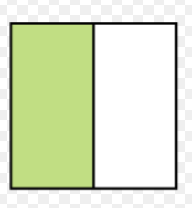1/2

100

Is 56 less than, greater than or equal to 60?

56 < 60

200

What column is the 7 in 87?

The one's place

200

23+10

33

200

20-10

10

2002/4

200

Is 80 less than, greater than or equal to 10?

80 > 10

300

What column is the 6 in 678?

The hundreds place

300

34+40

74

300

14-11

3
300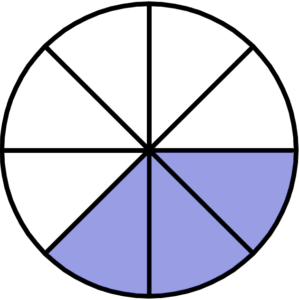3/8

300

Is 20 less than, greater than or equal to 20?

20 = 20

400

What column is the 3 in 523?

The one's place

400

100+20

120

400

12-6

6

400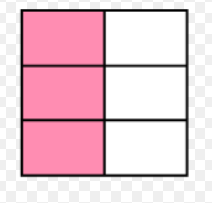3/6

400

Is 200 less than, greater than or equal to 30?

200 > 30

500

What column is the 3 in 1,309?

The hundreds place.

500

300+50

350

500

30-20

10

500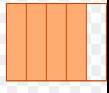4/5

500

Is 190 less than, greater than or equal to 195?

190 < 195

600

What column is the 4 in 4,568?

The thousands place.

600

610+20

630

600

50-30

20

600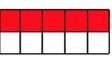5/10

600

Is 840 less than, greater than or equal to 500?

840 > 500

700

What column is the 7 in 6,790?

The hundreds place.

700

1,000+10

1,010

700

100-20

80

700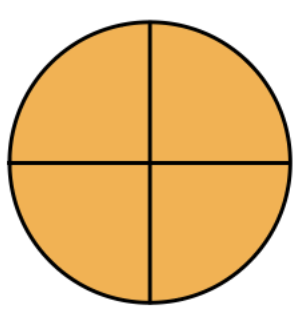4/4

700

Is 900 less than, greater than or equal to 900?

900 = 900

800

What column is the 0 in 10,987

The thousand's place.

800

10,000+10

10,010

800

388-80

308

8003/8

800

Is 10,004 less than, greater than or equal to 10,190?

10,004 < 10,190

Click to zoom